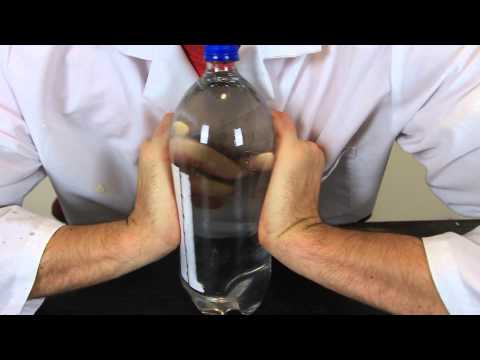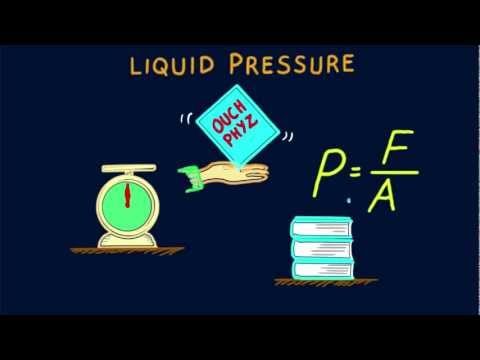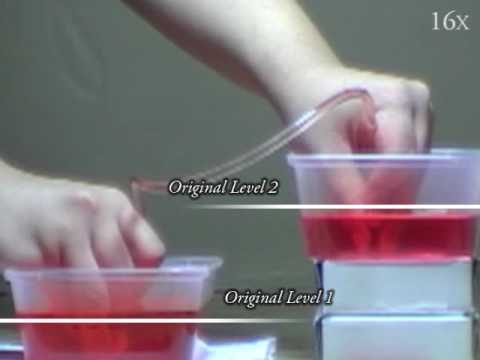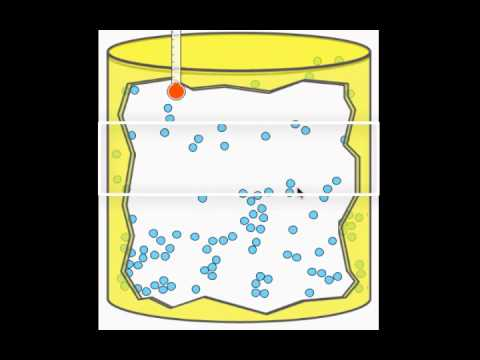## Pressure

Subject: Science

#### Overview

Pressure (P) is defined as the force (F) applied per unit area (A) perpendicularly. The pressure exerted by air is called atmospheric pressure. The pressure exerted by liquid is called liquid pressure. This note presents a brief information about pressure, atmospheric pressure and liquid pressure.
##### Pressure

Pressure (P) is defined as the force (F) applied per unit area (A) perpendicularly. It is measured in Pascal i.e. Newton per meter square.

Measurement of Pressure

Solution,

Let us consider a body having a cross-sectional area (A) exerting the pressure (P) by the force (F).

Now,

The pressure is directly proportional to the force.

That is, p∝ F...........(a)

Also,

The pressure is inversely proportional to the area.

That is, p∝ 1/A....... (b)

Again, combining equation (a) and (b)

P F/ A

Or, P = k x F / A (where k is proportional constant having value 1)

Or, P = 1 x F/ A

Or, P = F/ A

Hence, P = F/ A proved.

#### Atmospheric pressure

The Earth is surrounded by the thick layer of air which is known as atmosphere. It is extended up to 10,000km from sea level. The air has also mass. So, it exerts a certain pressure on the earth. The pressure exerted by air is called atmospheric pressure. It is exerted equally in all directions. At sea level, atmospheric pressure is 76 cm of mercury or 760 mmHg. It reduces with increase in height above sea level. Changes in atmospheric pressure bring about changes in weather. The nose of some people starts bleeding at high mountains because of the decrease in air pressure outside and more pressure inside our body.

Activity 1

Air exerts pressure on all sides.

1. Take a glass filled with water.
2. Cover the glass with cardboard or thick paper in such a way that no air passes in or out.
3. Invert the glass by placing one hand on the cardboard and the other at the bottom of the glass.
4. After pressing the board remove the hand from the board.
5. Now see what happens.

You can see that the cardboard does not fall. The cardboard does not let the water fall from the glass. The pressure exerted by air holds the cardboard without any external agent.

Importance of Atmospheric pressure

Due to atmospheric pressure, we are able to do various activities. Some of the activities done by atmospheric pressure are:

1. To fill ink in the pen.
2. To fill the air in the tire of the bicycle by the pump.
3. To fill medicine in the syringe.
4. To make the water pump.

Brainstorming question

An empty glass if inverted straightly doesn't sink. Why?

Activity 2

Air occupies space.

1. Take a glass filled with water.
2. Put the straw in the glass and suck the pipe.
3. Now see what happens.

When you suck the pipe there will be the low air pressure in the pipe. Due to atmospheric pressure water enters into the pipe and a person sucking the pipe gets water in his/her mouth. Likewise, while filling ink on pen and filling the syringe of medicine there will be the difference in the inner and atmospheric pressure. So, the pen gets filled with ink and syringe gets filled with medicine.

#### Liquid pressure

Matter occupies space and has weight. It can exert pressure as well. The pressure of a body depends on the weight as well as on the surface area occupied by it. The liquid is a state of matter. Since the matter has weight and occupies space, the liquid also occupies space and has weight. A body which has weight can exert pressure. Therefore, the liquid can also exert pressure.

The pressure exerted by the liquid is called liquid pressure. The pressure in a liquid increases with depth, but at the same depth, the pressure is same in all the directions. Liquid pressure depends on the height of the liquid, density of the liquid and the acceleration due to gravity.

Measurement of Pressure of Liquid

Let's consider the liquid having density "d" is filled into the vessels having a cross-sectional area of "A" meter square. The liquid is filled up to height 'h'. If 'g' is the acceleration due to gravity and 'V' be the volume of liquid then we have,

Pressure(p) = $\frac{Weight \: of\: the\: liquid(w)}{Cross- sectional\: area\: of\: vessel(A)}$

p = $\frac{w}{A}$

p = $\frac{ mass(m) \times acceleration\: due\: to\; gravity(g)}{A}$ ( $\because$ w = mg)

= $\frac{density(d) \times volume(v) \times g}{A}$ ( $\therefore$ m = d $\times$ v)

= $\frac{d \times v \times g}{A}$

= $\frac{d \times A \times h \times g}{A}$ (v = A $\times$ h)

$\therefore$ p = dhg

Activity 3

Liquid pressure increases with the depth.

1. Take a cylindrical tin can. Remove its lid.
2. Make three holes of the same width at three different levels from the top.
3. Cover the holes with the help of cello tape.
4. Fill the Cylindrical can with water up to the brim.Source: spmphysics.onlinetuition.com.my Fig: Liquid pressure

Activity 4

The liquid pressure is same in all directions at the same depth.

1. Take an empty tin can.
2. Make five holes of the same width at the same depth all around it.
3. Cover the holes with cello tape.
4. Fill the can with water.
5. Lift it with some string so that its bottom is horizontal.
6. Remove the cello tape from the holes.
7. The water rushes out through holes and falls at the same distance from the bottom of the can on all sides. This indicates that water rushes out through the holes with the same pressure.
8. This proves that water pressure (the pressure exerted by water) at the same depth, is the same in all directions.

Example: The weight of a box is 100N and its base area is 2-meter square. Calculate the pressure exerted on the ground by the box.

Solutions,

Weight (w) = 100N

Area (A) = 2 m

Pressure (P) =?

We know,

P = F/ A

Or, P = F/ A

Or, P = 100/ 2

Density

Density is a physical property of a matter. It can be defined as a ratio of mass per unit volume. The qualitative definition can be the measure of the relative heaviness of an object with constant volume. For example, rock is denser than the piece of paper though they occupy the same volume (differ in the quantity of mass occupied in the same volume).

The formula for measuring density is density= mass/volume. Unit of density is kg/m3.

Relative density

Relative density is the ratio of the density of the substance to the density of water at 4 degree Celsius of the same volume is called relative density. Relative density does not have a unit. According to the density of the liquid, upthrust differs. If the liquid has higher density,then upthrust exerted by it increases. That is why an egg does not float on pure water but floats on salt water. With the addition of salt, we increase the density of water. Due to higher density, the egg floats.

Floating and Sinking

Some substance float whereas some objects sink in water. Generally, the substance which has the higher density than that of water sinks in water and substance with lower density floats in water.

Activity 5

Floating and sinking depend on density

1. Take a beaker with clean water.
2. Take an egg and put that gently on the water.
3. Now mix salt in water until the egg floats on water.

When an egg is kept in water egg sinks in water because the density of egg is greater than that of water. The egg floats on after adding salt in water because salt increases the density of water and density of water becomes more than that of an egg, so the egg floats on water.

##### Things to remember
• Pressure (P) is defined as the force (F) applied per unit area (A) perpendicularly.
• Pressure is measured in Pascal which is Newton per meter square.
• Atmospheric pressure : The pressure exerted by air is called atmospheric pressure.
• Liquid pressure : The pressure exerted by liquid is called liquid pressure.
• Pressure = F/ A
• F = m x g
• liquid pressure (P) = d × g × h
• It includes every relationship which established among the people.
• There can be more than one community in a society. Community smaller than society.
• It is a network of social relationships which cannot see or touched.
• common interests and common objectives are not necessary for society.
##### Videos for Pressure##### Atmospheric Pressure - Science Experiment##### Bill Nye: The Science Guy - Pressure (Full Episode)##### Hewitt-Drew-it! PHYSICS 58. Liquid Pressure##### Pressure in a Liquid: Demonstration 1##### Why does air pressure decrease at higher altitudes?

The pressure is the force exerted per unit area. The SI unit of pressure is Newton per meter square.

The pressure exerted by air or atmosphere is called atmospheric pressure.

As we know that a sharp nail covers less area but the blunt one covers more area. Since the area is less in the sharper nail so the pressure is more but the blunt one has less pressure as it covers more area, so a sharp nail pierce a wooden block easily whereas a blunt one does not.

 S.N. Air pressure Atmospheric pressure 1. It is measured by air gauge. It is measured by barometer. 2. Air pressure is local pressure, such as in a tire or balloon. Atmospheric pressure is the weight per unit area of 5 miles height of air piled up and acting at the Earth's surface. 3. Air pressure includes any air that is pressurized. Atmospheric pressure is the pressure of the air in the atmosphere.

 S.N. Force Pressure 1. Force is the product of mass and acceleration. Pressure is the force acting per unit area. 2. F= P x A P = F/A 3. Unit=N i.e Newton Unit= Pa i.e Pascal

The pointed heel of a girl has less surface area than the leg of the elephant. As pressure is inversely proportional to the area, so the pointed heel of a girl exerts more pressure.

The foundation of building is made wider than walls in order to reduce the pressure exerted by its ceiling on the base.

Truck and buses have back wheels in pairs to reduce the pressure exerted by heavy loads on the wheels of buses and trucks.

Solution,

\begin{align*} \text {Given,} \\ \text {area} \:(A) = 0.1\:m^2 \\ \text {pressure}\:(p) = 1000\: Nm^2 \\ \text {force} \:(F) = ? \\ \text {we know,} \\ P &= \frac FA \\ \text {or,}\: F &= P\times A \\ &= 1000 \times 0.1 \\ &= 100\: N \\ \therefore \text {The force applied is } 100\: N\end{align*}

Solution,

\begin{align*} \text {Given,} \\ \text {Depth} \: (h) = 0.5\:m \\ \text {pressure} \:(p) = 5000\: N/m^2 \\ \text {Acceleration due to gravity} \:(g) = 10 m/s^2 \\ \text {Density} \:(d) =\: ? \\ \text {We know that,} \\ P &= h\times d\times g \\ \text {or,}\: 5000 &= 0.5 \times d\times 10 \\ \text {or,}\: 5 \times d &= 5000 \\ \text {or,}\: d &= \frac {5000}{5} \\ \therefore \: \text {Density is equal to} \: 1000\: kg/m^3 \end{align*}

Solution,

\begin{align*} \text {Given,} \\ \text {Force}\: (f) = 40\: N \\ \text {Pressure}\: (p)\: =\: 8000 \:N/m^2 \\ \text {Area} \:(A)\: =\: ? \\ \text {We know that} \\ \text {Pressure}\:(p) &= \frac FA \\\text {or,} \: A &= \frac FP \\ \text {or,} \: A &= \frac {40}{8000} \\ \therefore \text {The area is }\: 0.005\:m^2. \\ \end{align*}

Solution,

\begin{align*} \text {Given,}\:\text {Mass}\: (m)\: =\: 60\: kg \\ \text {Area}\: (A)\: =\; 120\: cm^2 \\ &= \frac {120}{100 \times 100} \ \\ &= 0.012\:m^2 \\ \text {Pressure}\: (P)\: =\:? \\ \text {Force}\: (F)\: &=\: m \times g \\ &=60 \times 10 \\ &=600\: N \\ \text {We know,}\\ P &= \frac FA \\ &= \frac { 600}{0.012} \\ \text {Now,} \\ \ &=5 \times 10^4 N/m^2 \\\text {His pressure on the floor is} \:5\times10^4 N/m^2. \\ \text {Now,} \\ \text {Area}\: (A)\: =\: 15000\: cm^2 \\ &=\frac {15000}{100 \times 100} \\ &=1.5 m^2 \\\text {Force}\: (F)\: =\: 600\: N\\ \text {Pressure}\: (P)\: &= \frac FA \\ &= 600/1.5 \\ &= 400 N/m^2. \\ \text {His pressure on the floor after sitting is} \: 400N/m^2.\\ \end{align*}

Barometer is an instrument used for measuring the atmospheric pressure. An Italian scientist named Torricelli invented this. It is made by one-meter long glass closed at its one end, which is taken and filled completely with mercury. Then, by closing the mouth of tube, it is inverted in a trough containing mercury. We can see that the level of the Hg in the glass tube decreases at first and finally becomes constant. The empty space above  the surface of Hg in the tube is collected in Tarrecellian vacuum.

Solution,

Let us consider a liquid in the beaker. Let 'A' be the area of the beaker and 'h' be the height upto which the liquid is filled. If 'd' be the density of the liquid and volume= A $\times$ h, then we have,

\begin{align*} P & = \frac {\text {weight of the liquid}}{ \text {base area of a container}} \\ \text {or,}\: P &=\frac WA \dots (i) \\ \text {or,}\: P &= \frac {m \times g}{A} \dots (ii) \\ \text {Here, weight of the liquid}\: (w)\: &= mg \\ \text {We can also say that,} \\P &= \frac {dwg}{ A} \dots (iii)\:\:\:\: [\text {since,}\: d =\frac mv \: \text {or} m=d \times v] \\ \text {or.}\: p &= d \times \frac {(A \times h)g}{ A} [since, v= A x h]
\\ \therefore P &= dhg,\: \text { proved.} \\ \end{align*}